# RD Sharma Solutions for Class 11 Chapter 13 - Complex Numbers Exercise 13.2

Problems related to imaginary quantities (the square root of a negative real number is called an imaginary quantity or an imaginary number), addition, subtraction, multiplication, division of complex numbers using its properties, the conjugate of a complex number, modulus and reciprocal of complex numbers are discussed in this exercise. Expert tutors at BYJU’S have formulated the solutions in a step by step manner where every student can understand the concepts clearly. Students can refer to RD Sharma Class 11 Solutions for Maths and can also download the pdf from the links given below.

## Download the pdf of RD Sharma Solutions for Class 11 Maths Exercise 13.2 Chapter 13 – Complex Numbers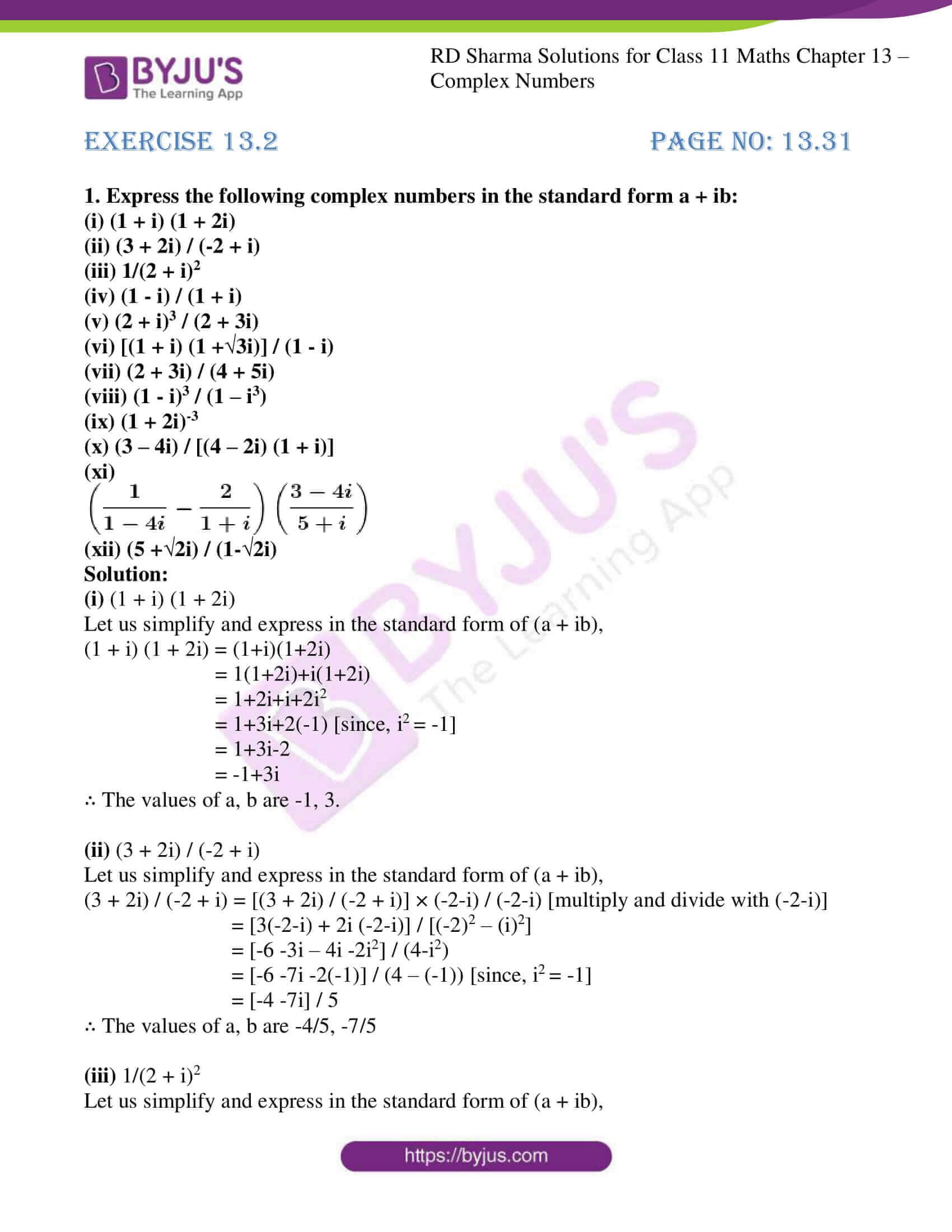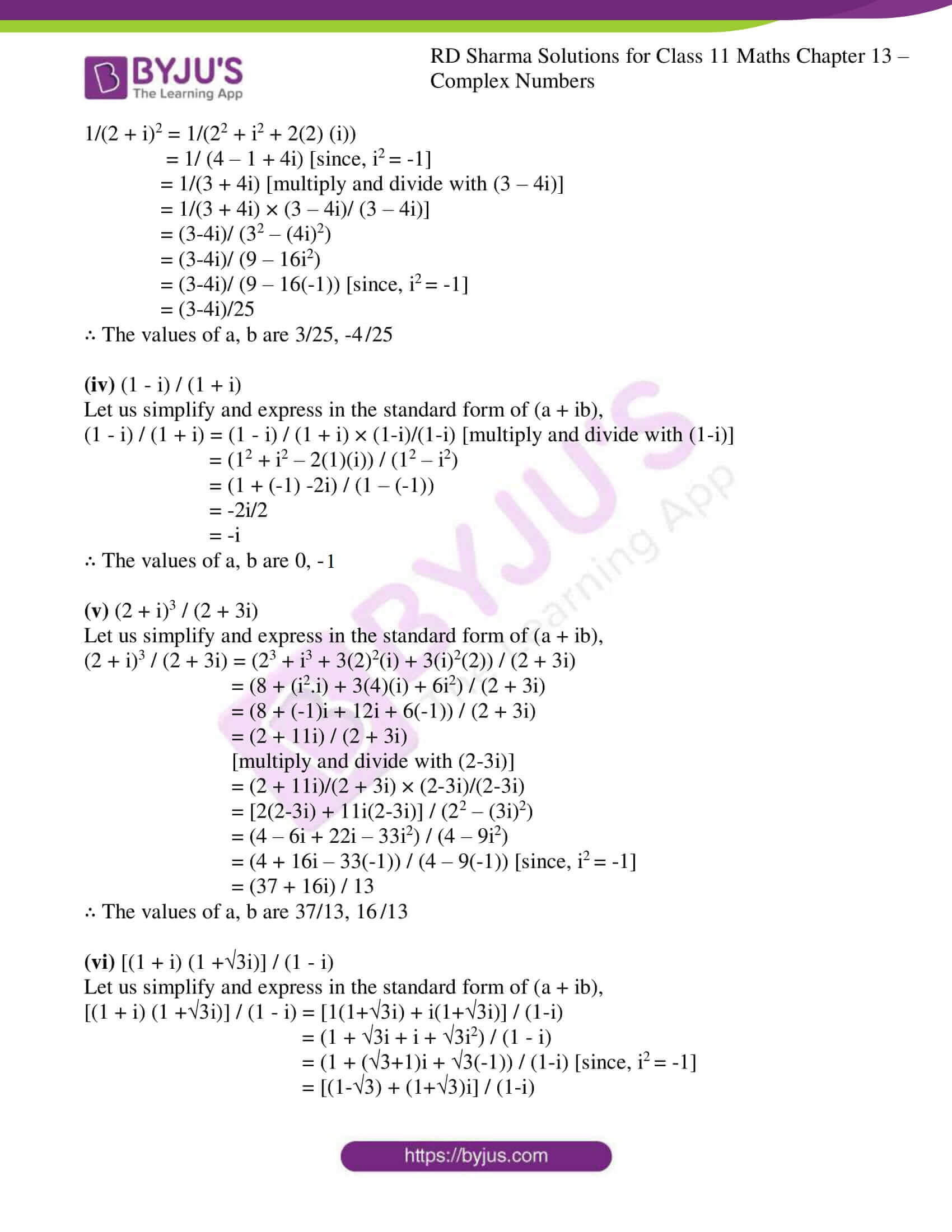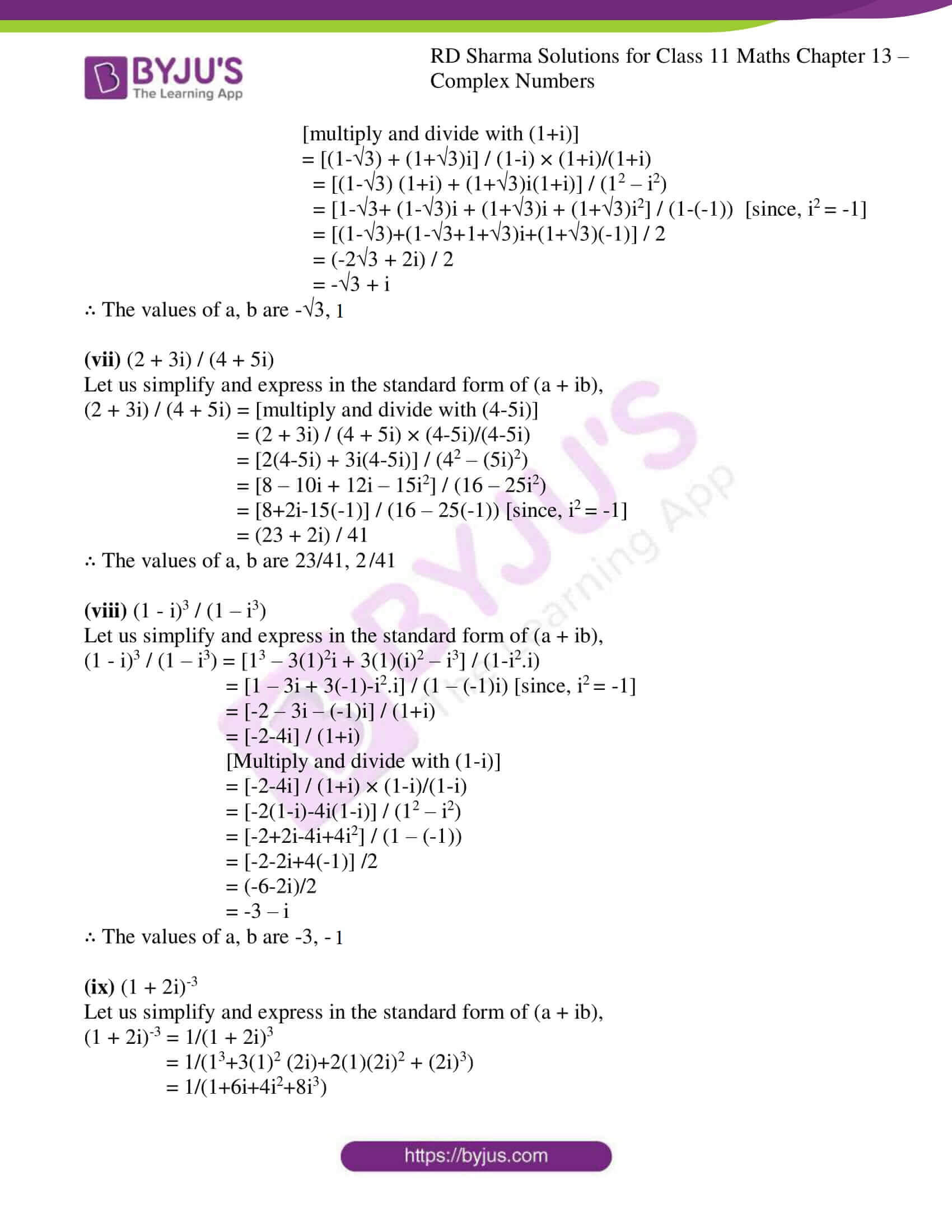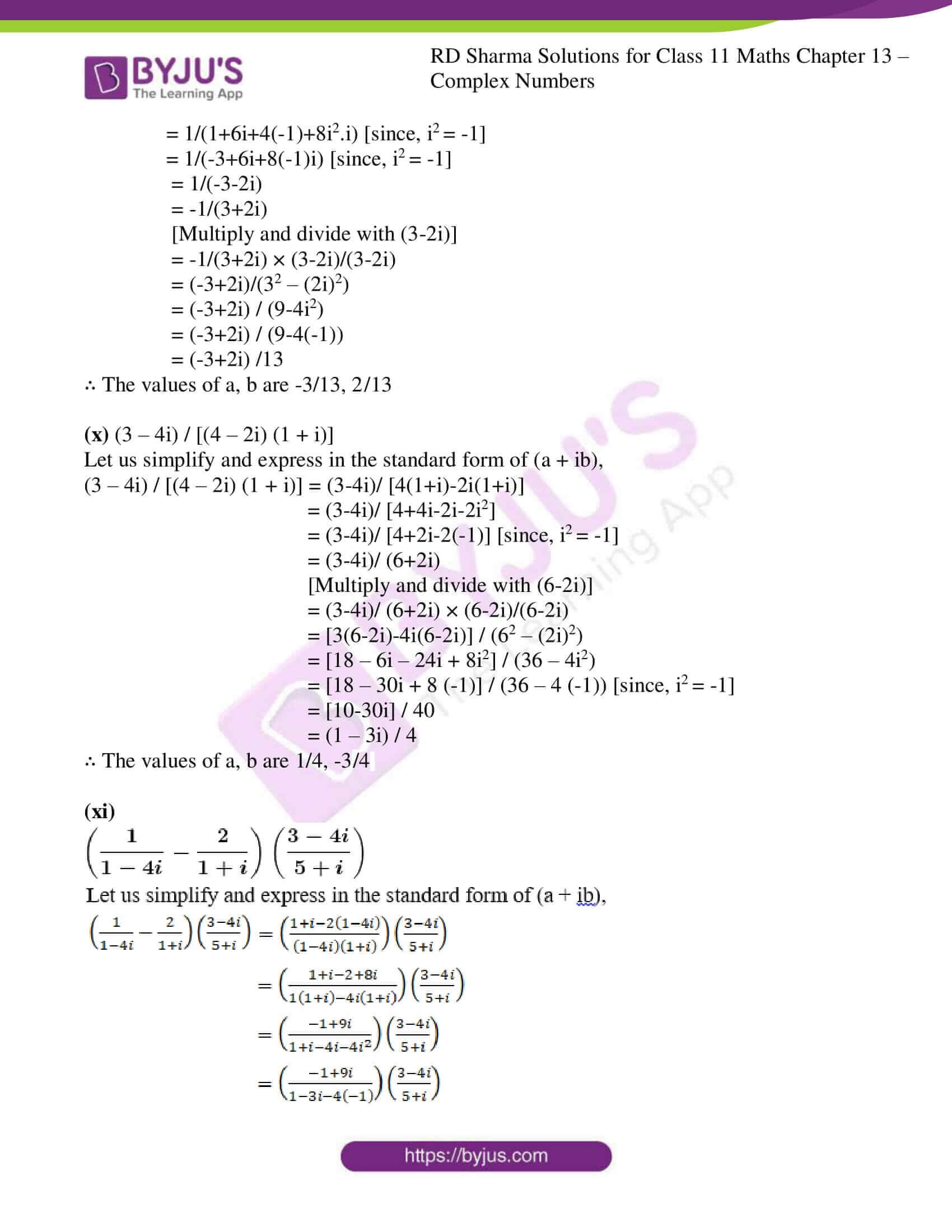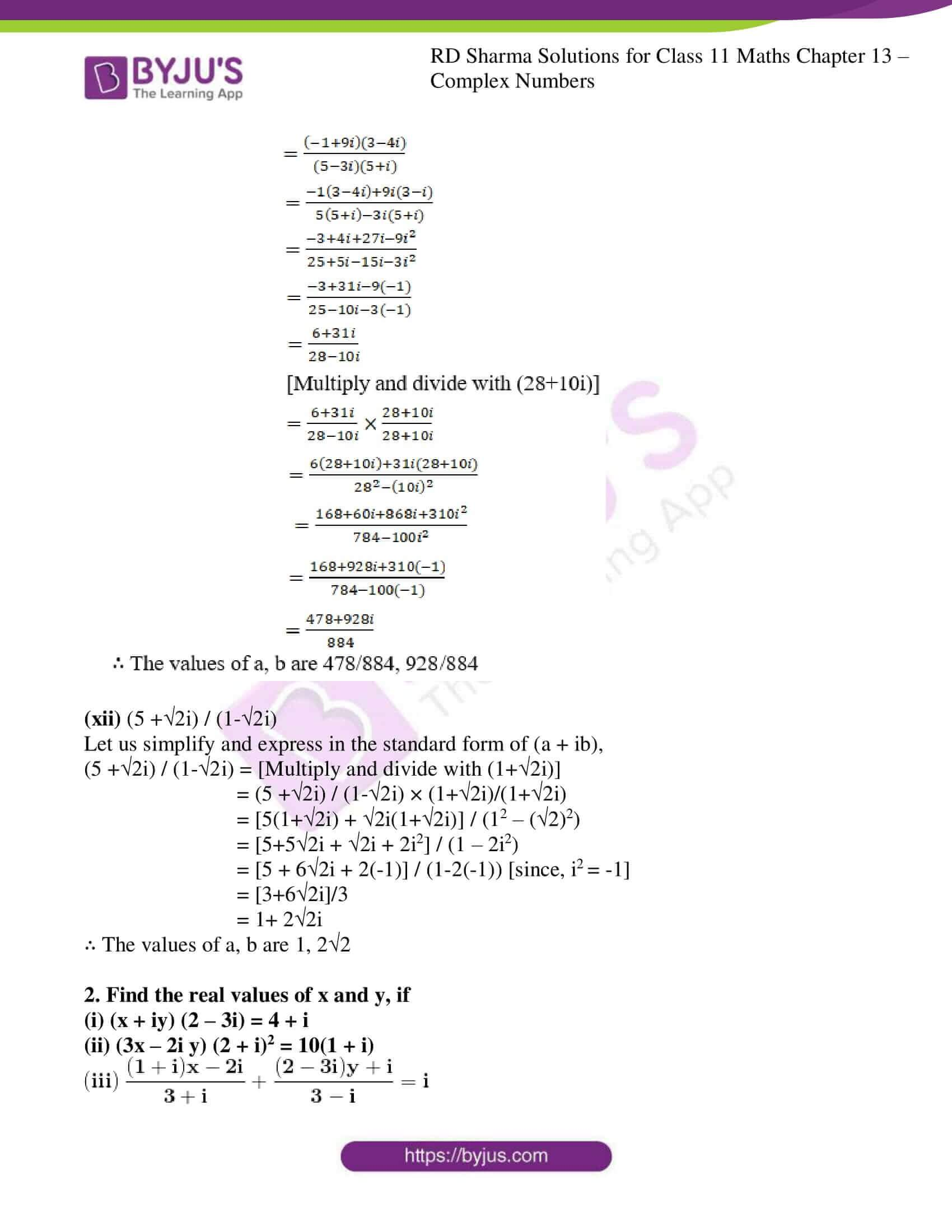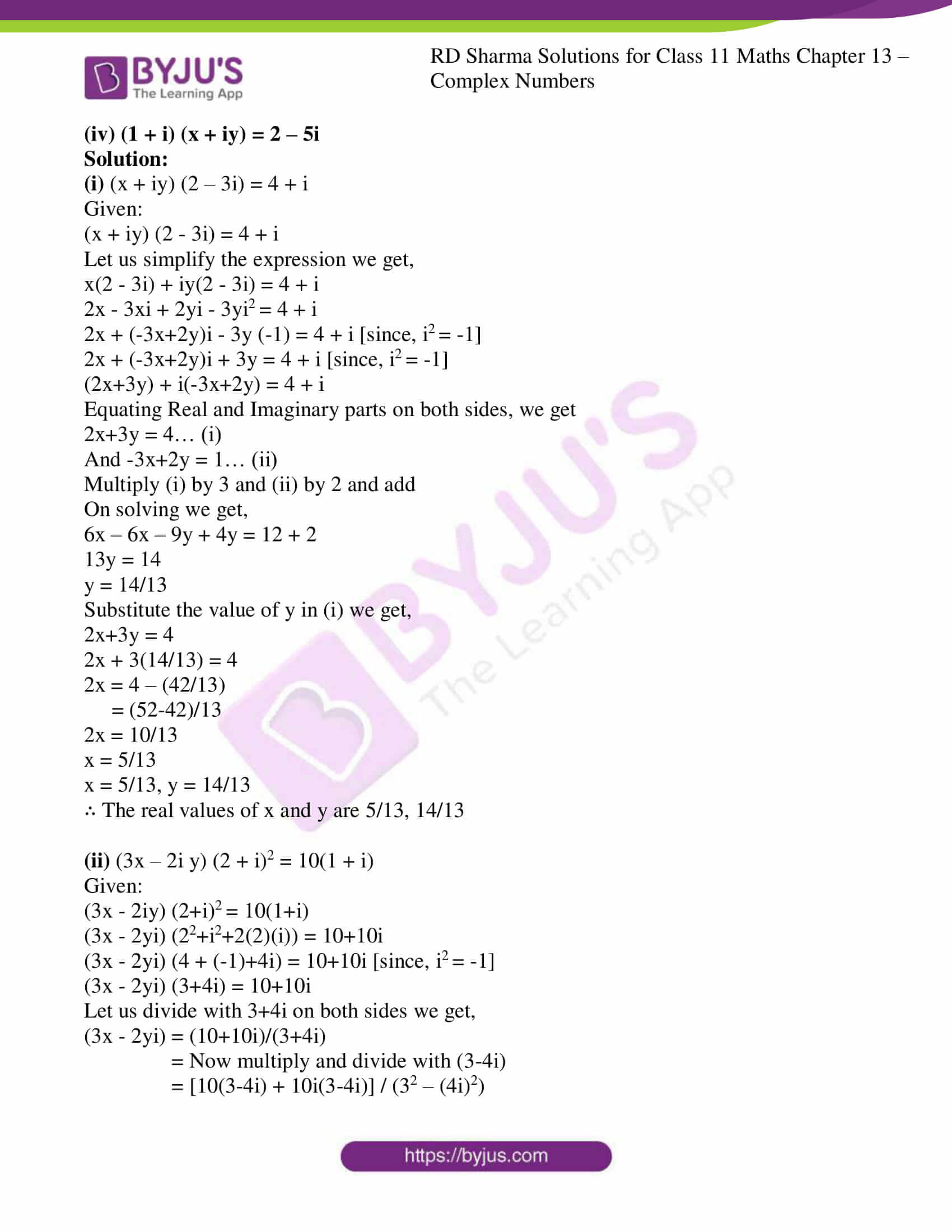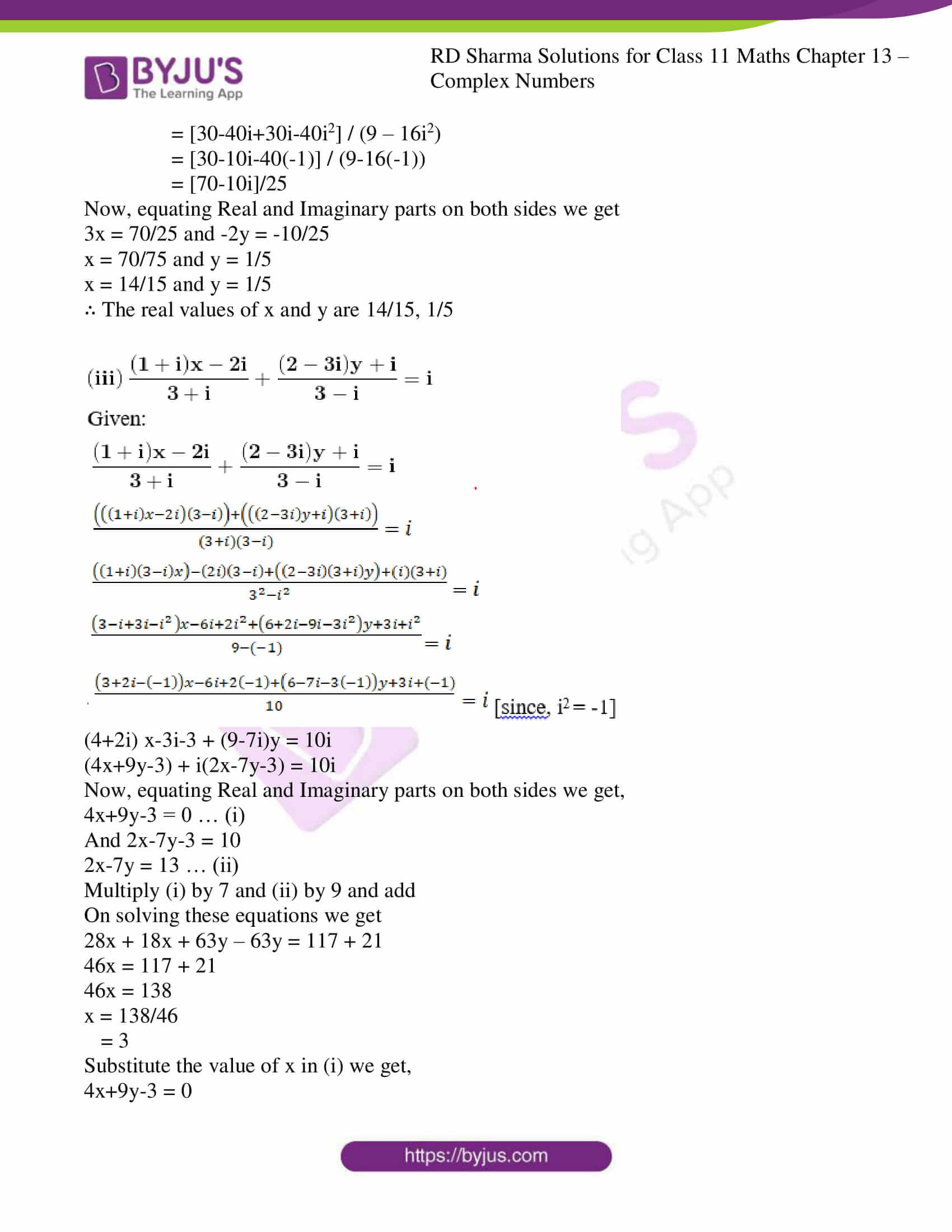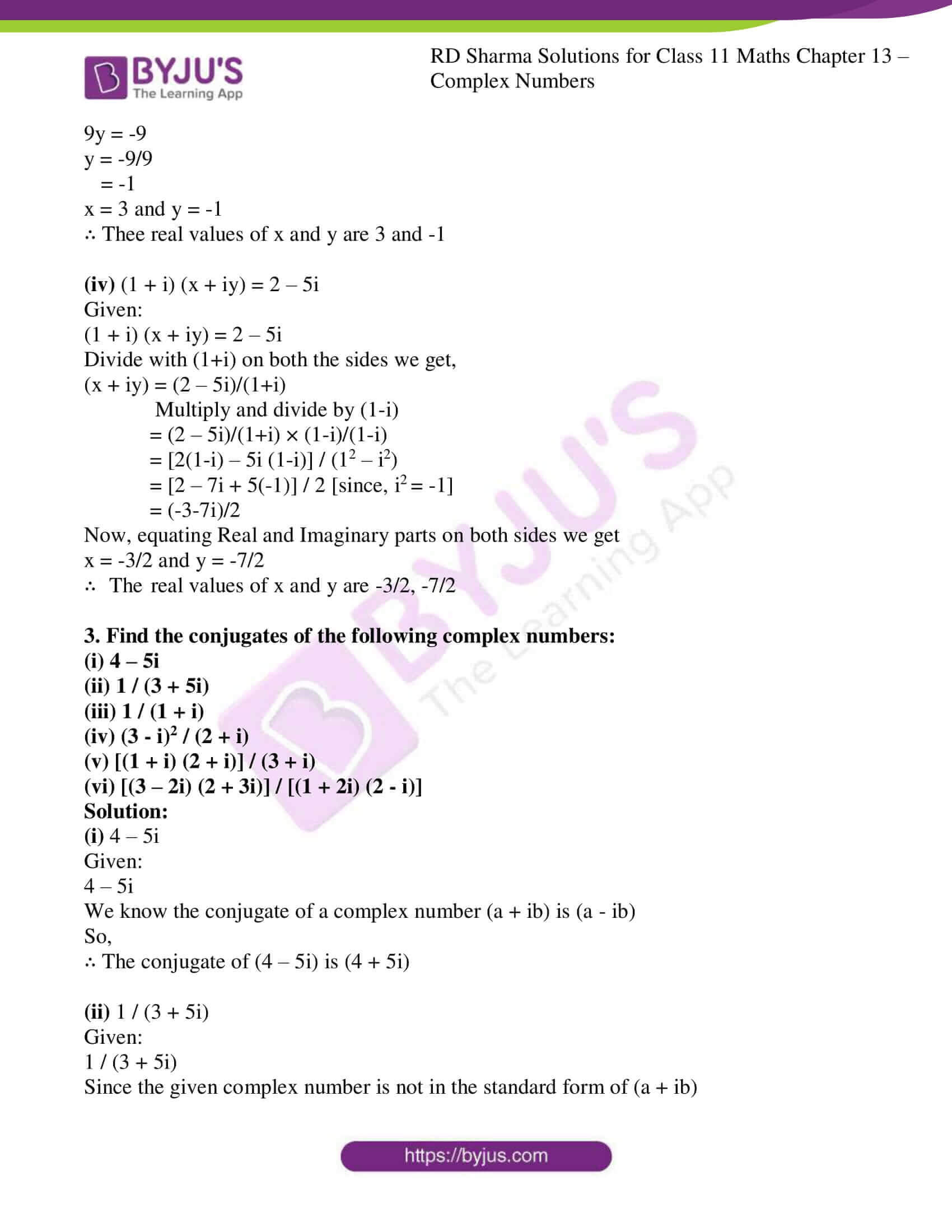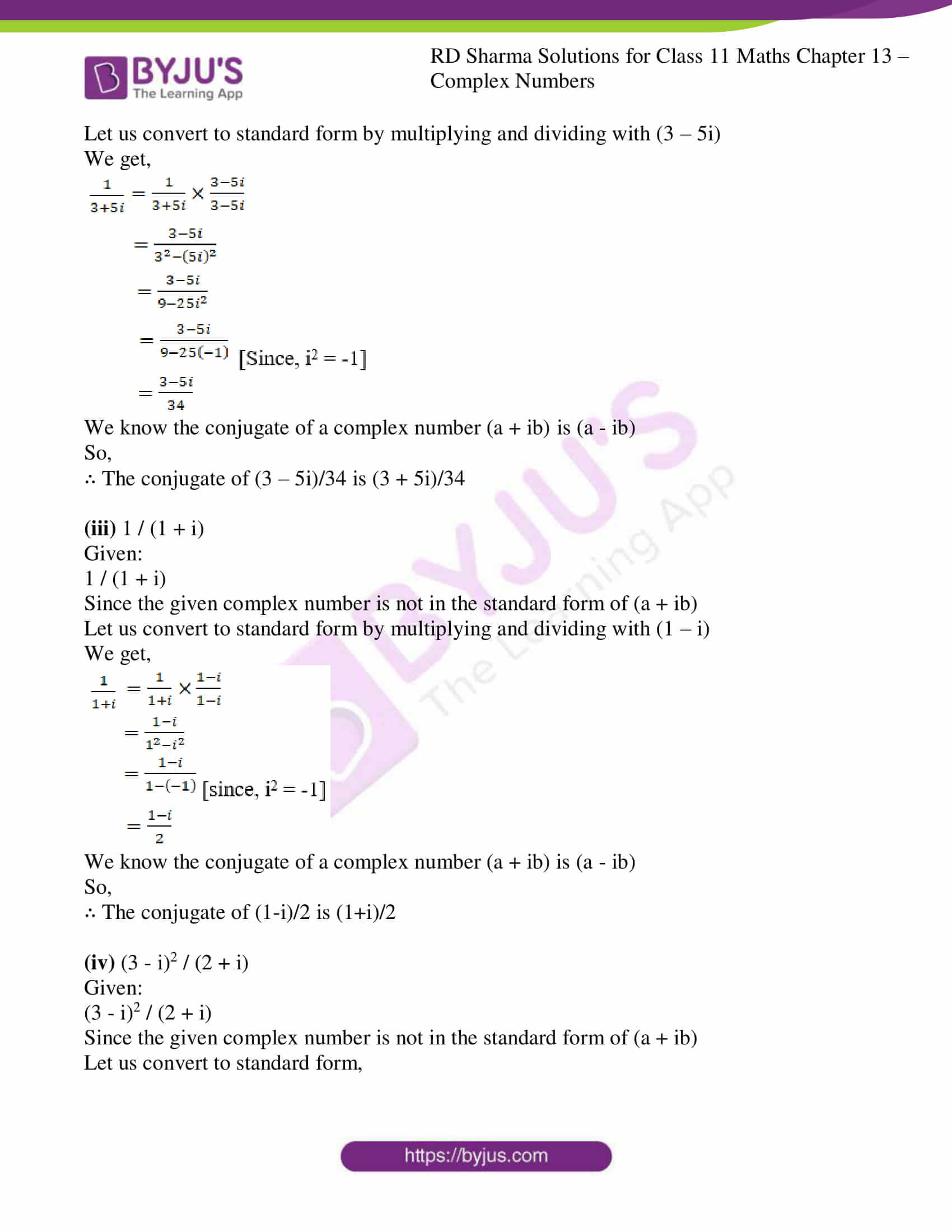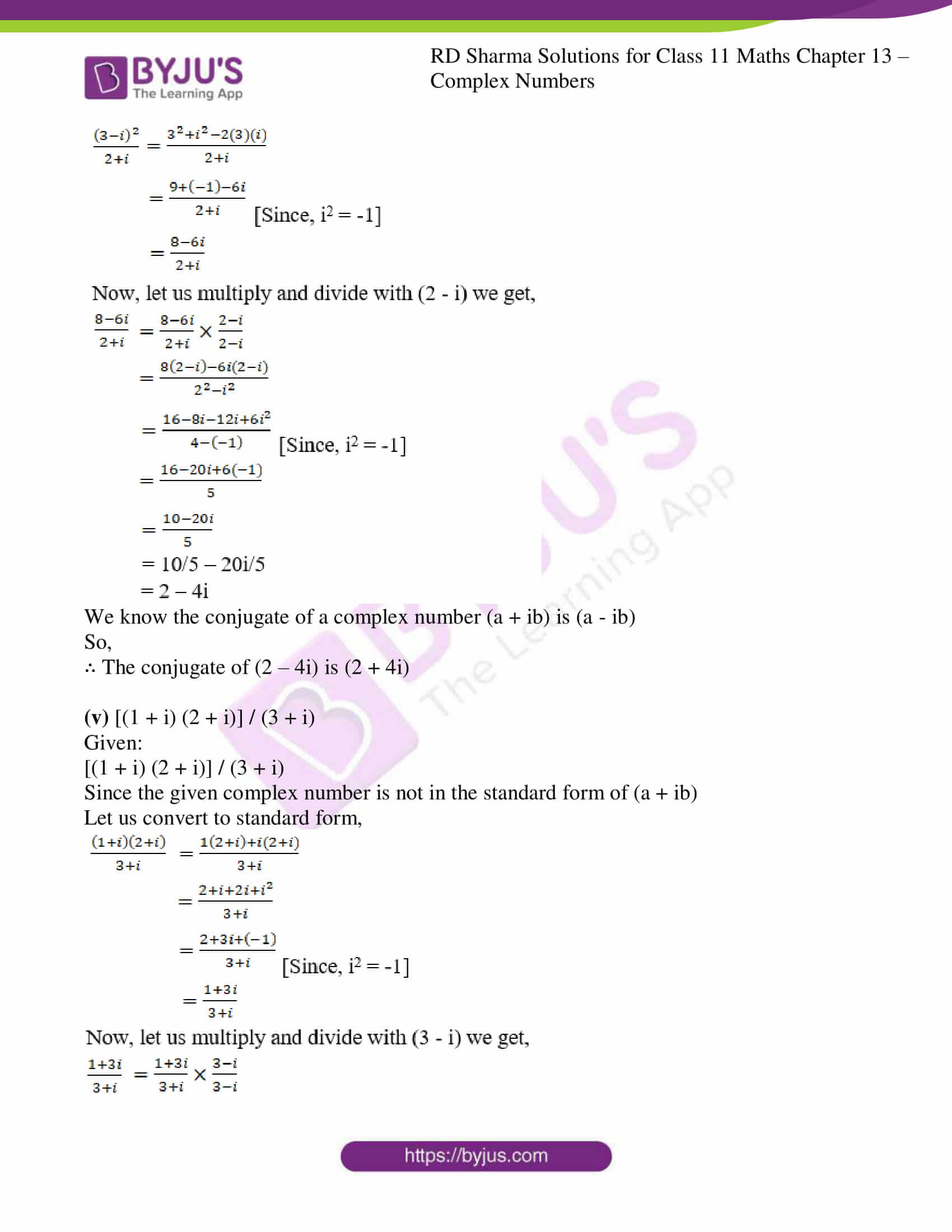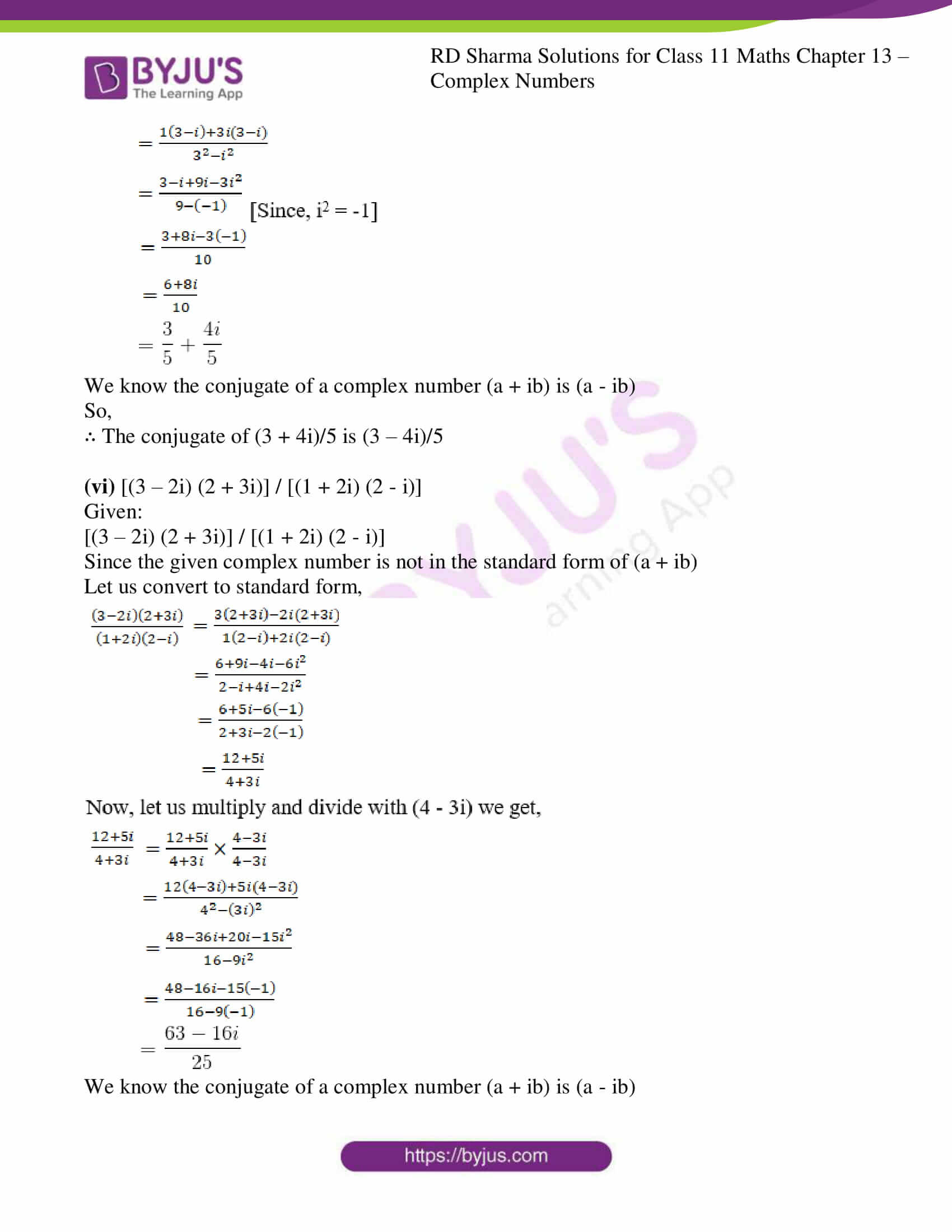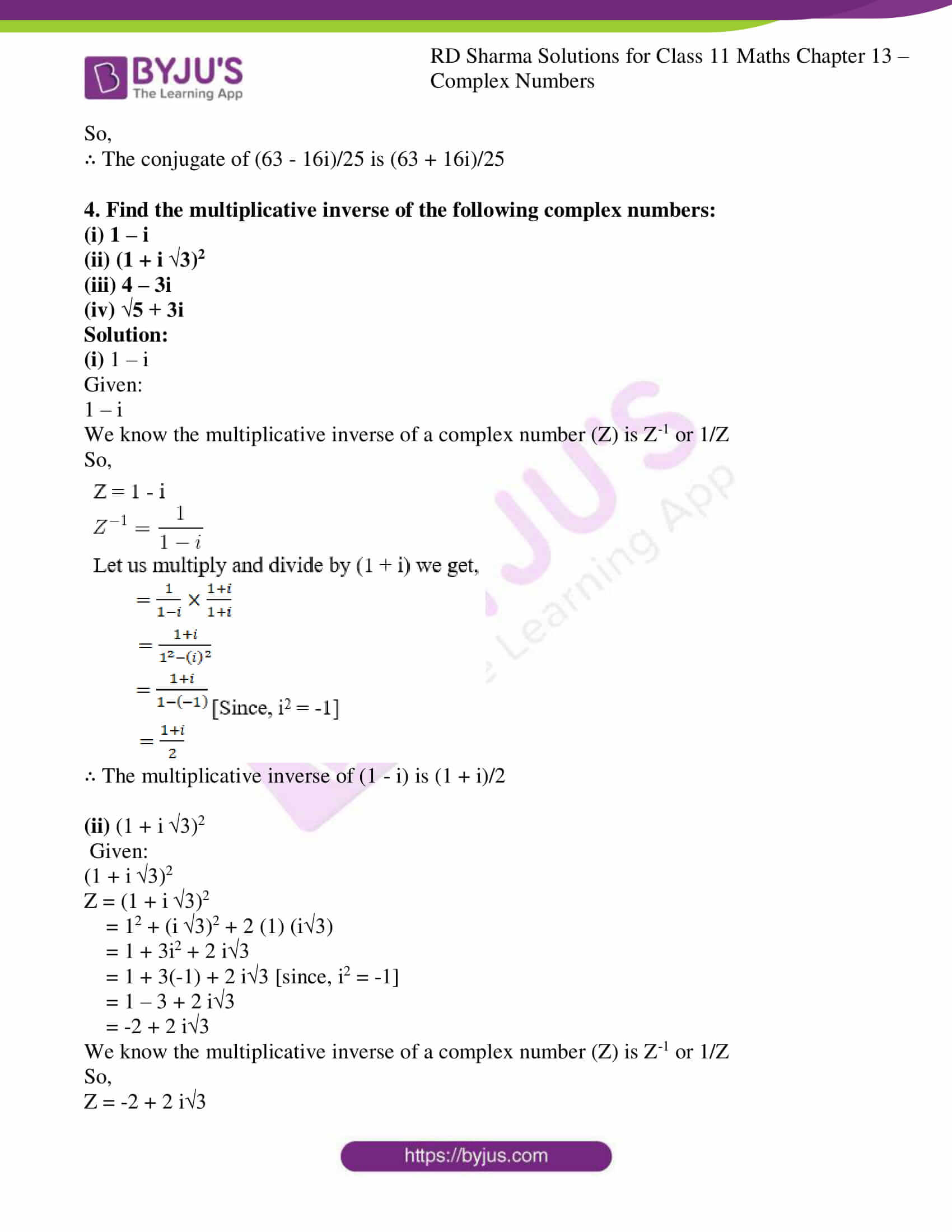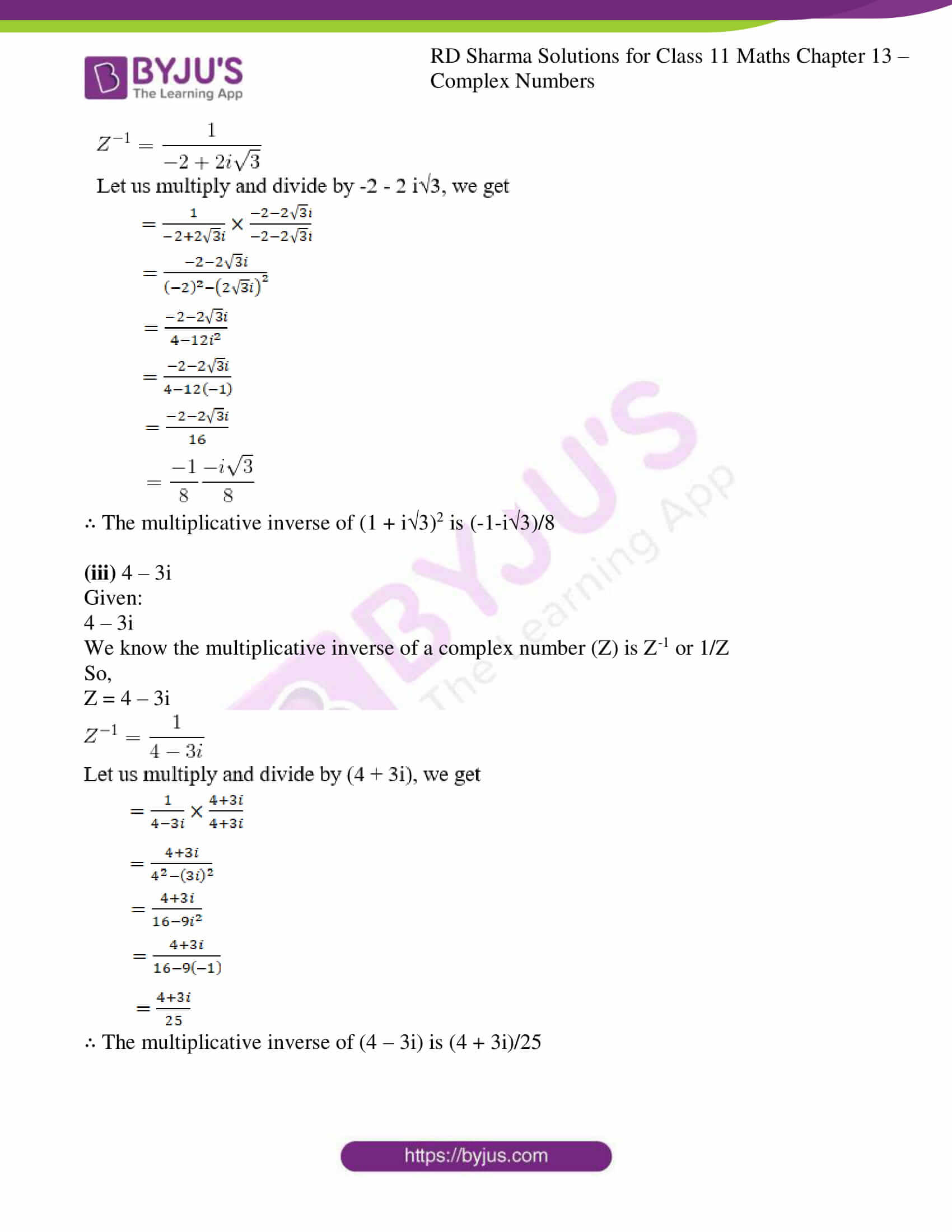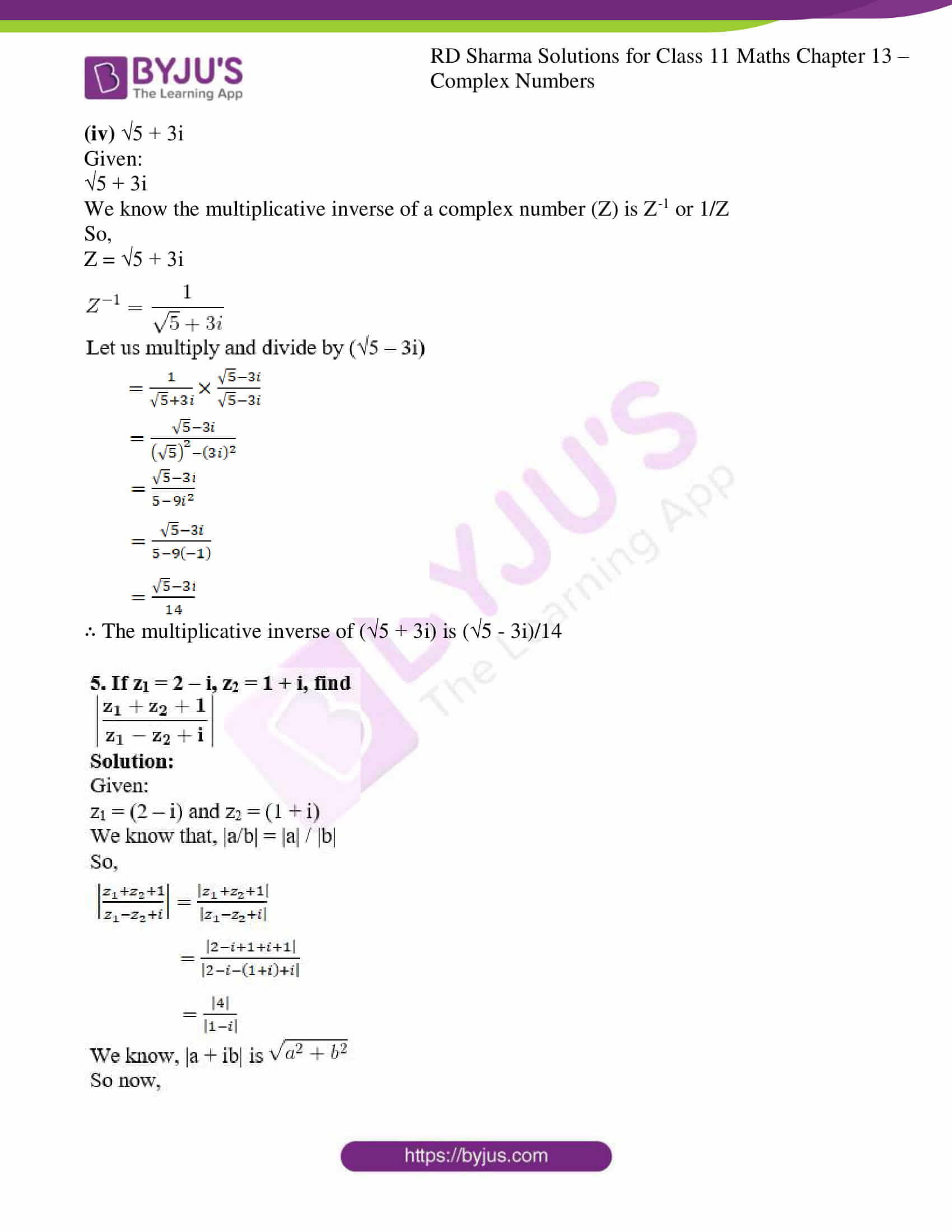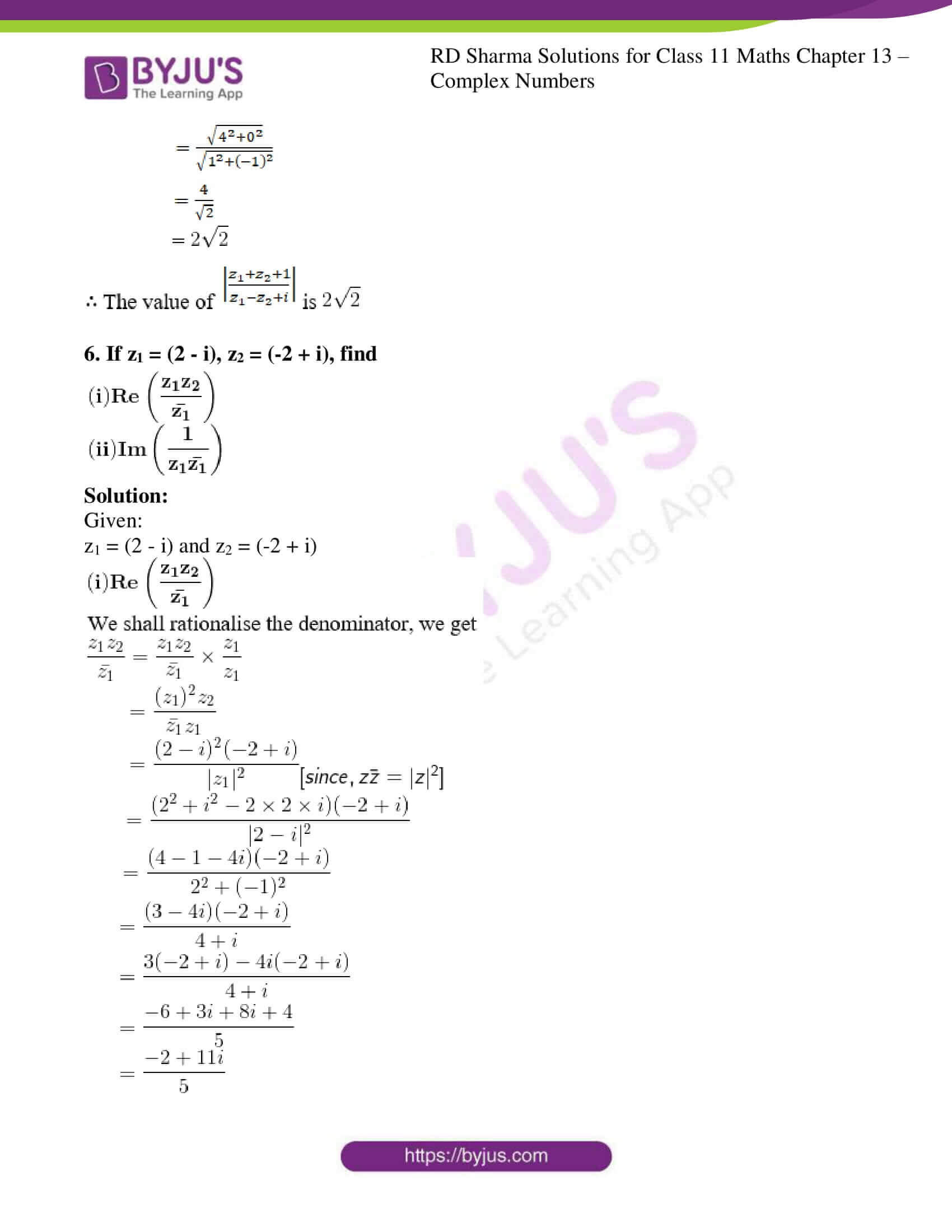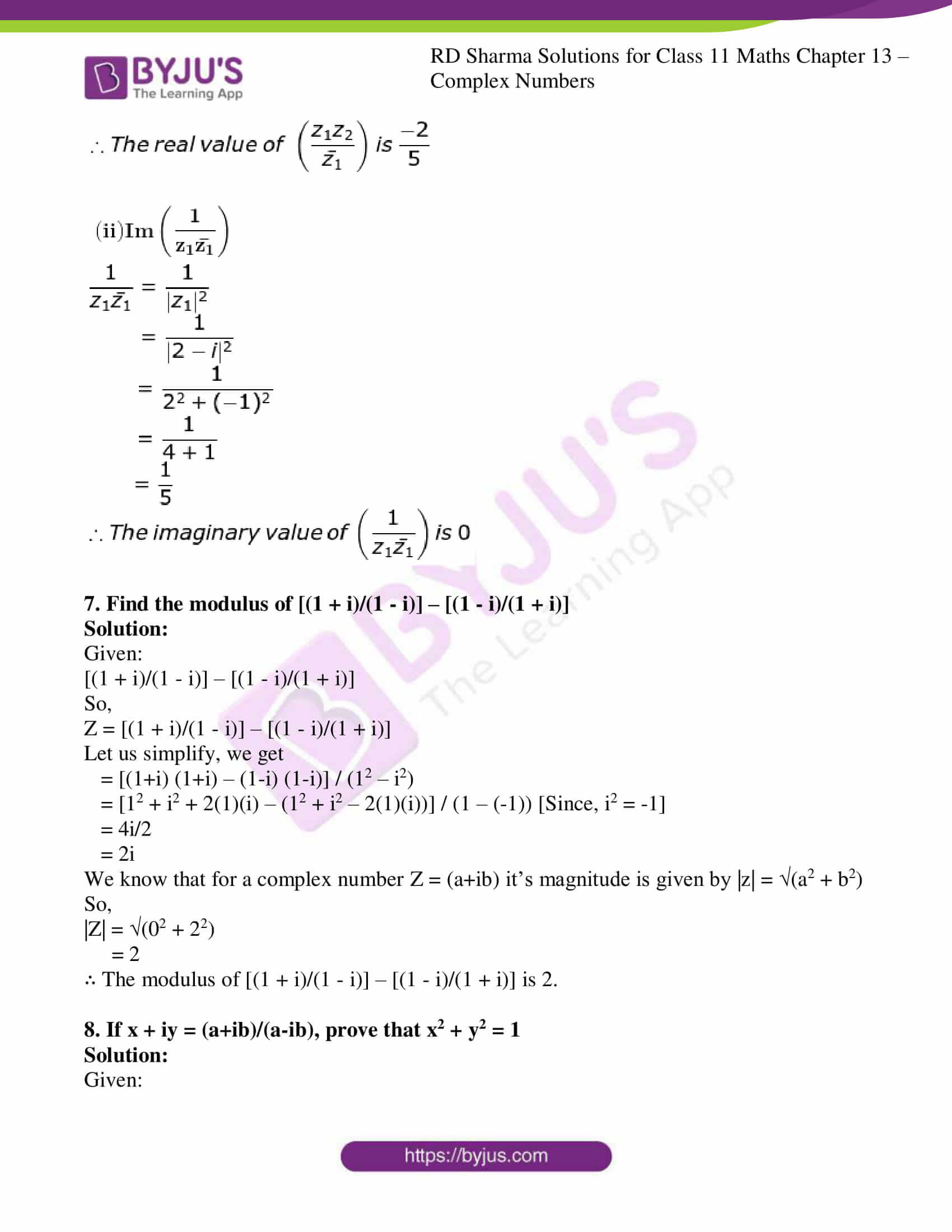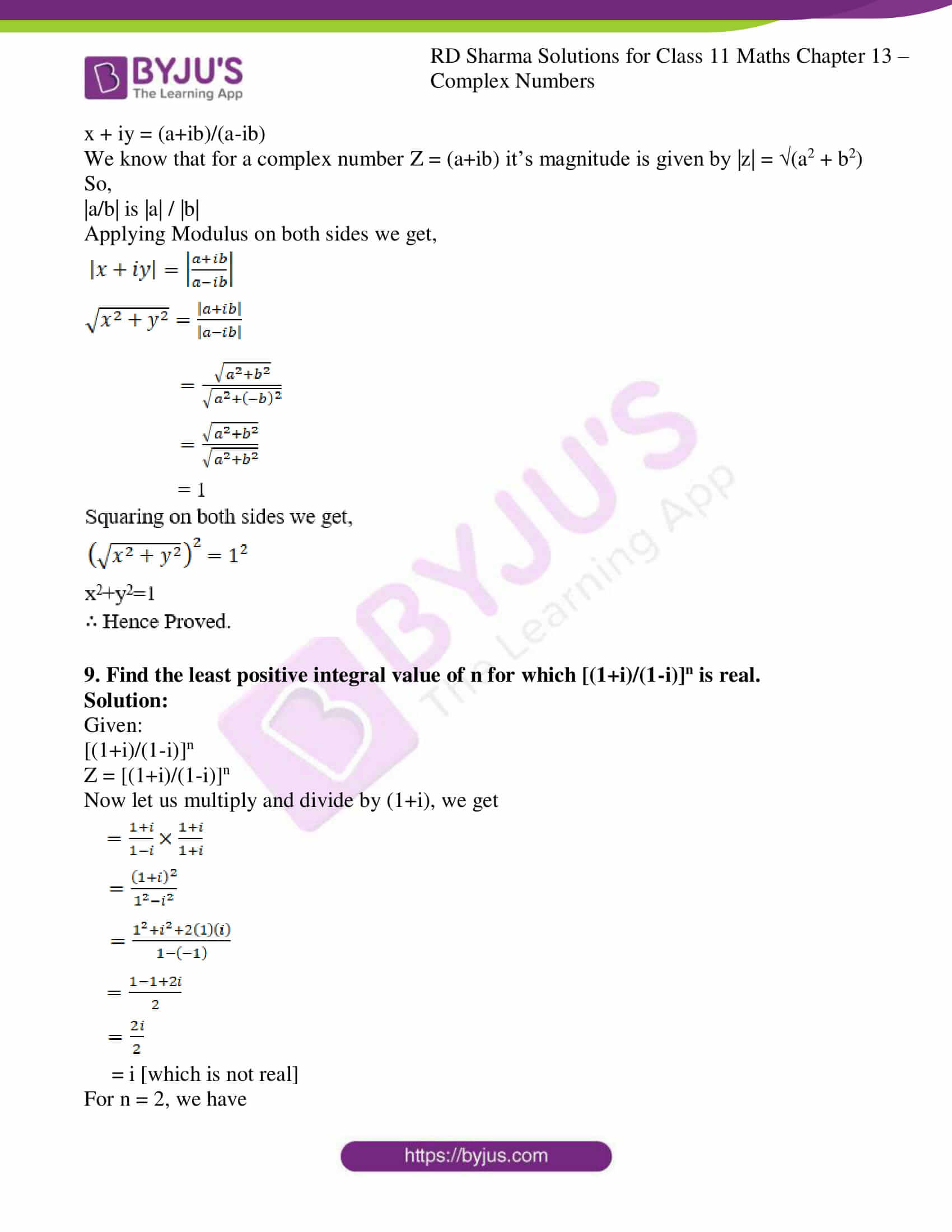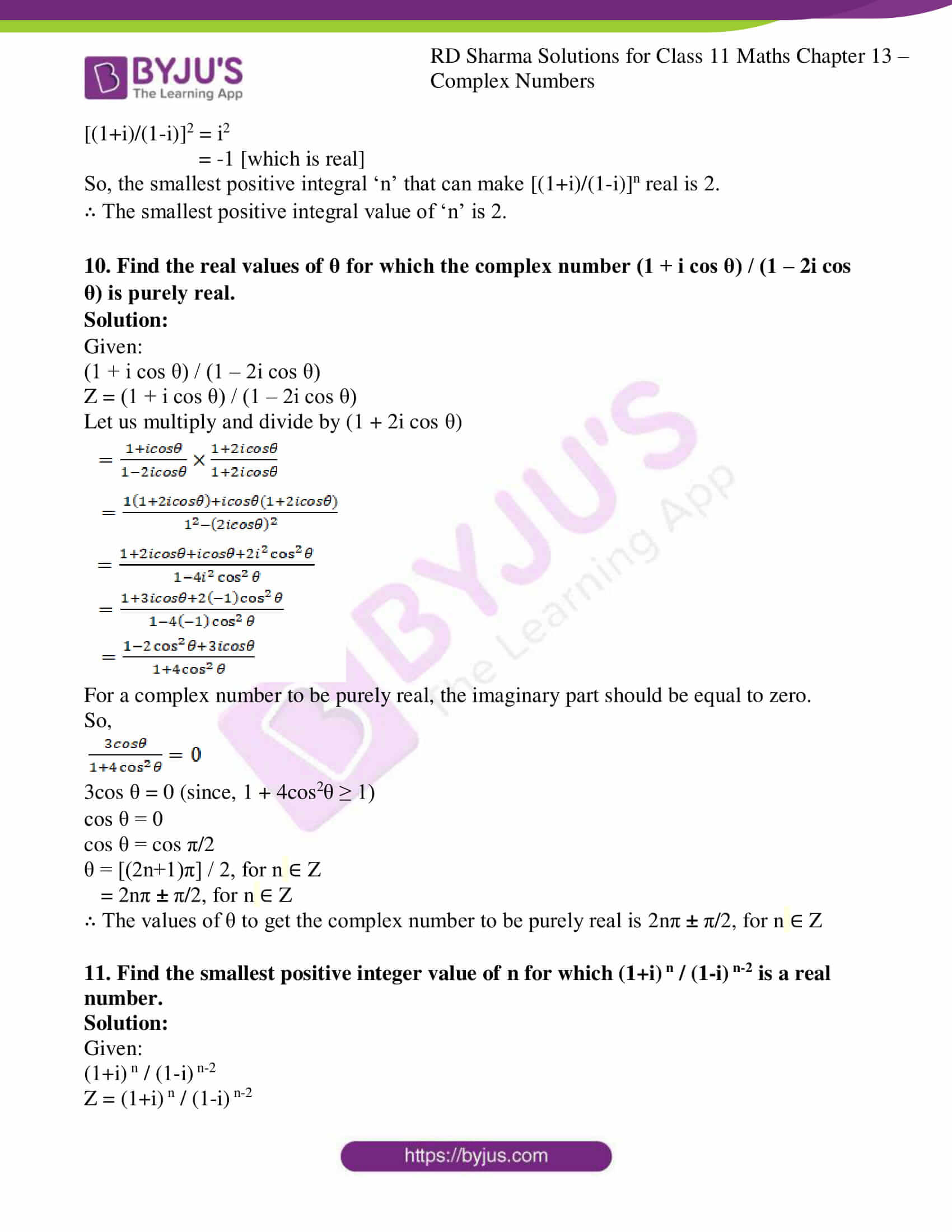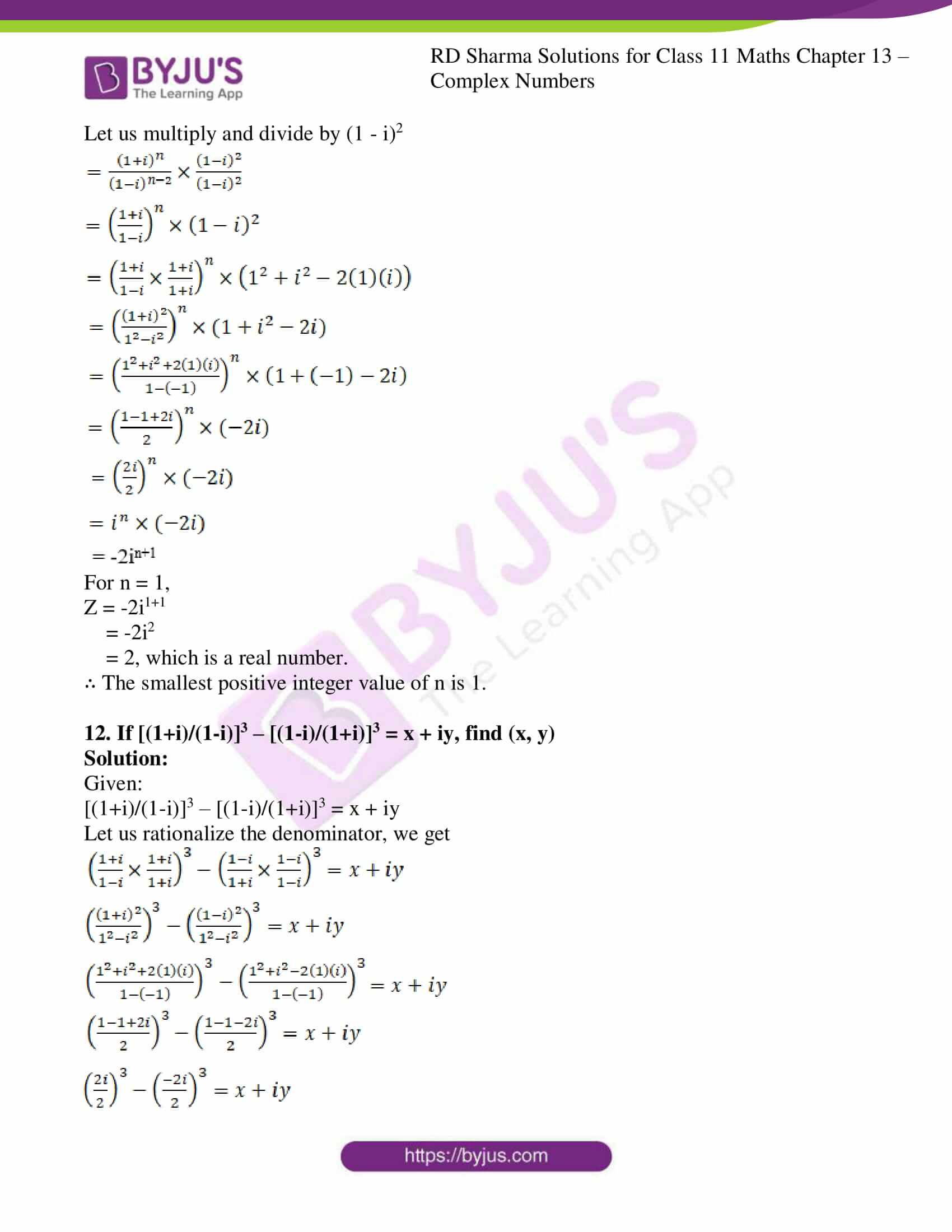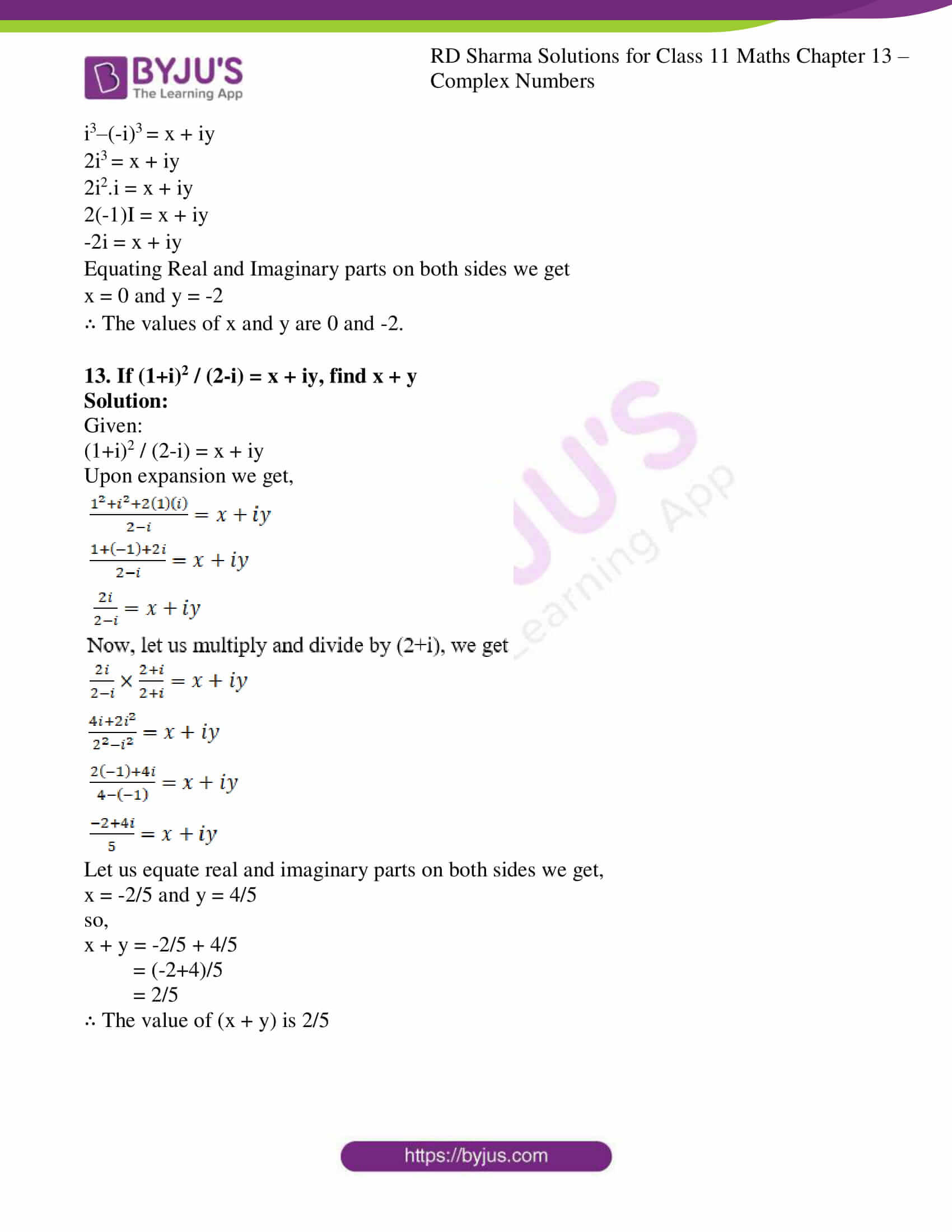### Also, access other exercises of RD Sharma Solutions for Class 11 Maths Chapter 13 – Complex Numbers

Exercise 13.1 Solutions

Exercise 13.3 Solutions

Exercise 13.4 Solutions

### Access answers to RD Sharma Solutions for Class 11 Maths Exercise 13.2 Chapter 13 – Complex Numbers

1. Express the following complex numbers in the standard form a + ib:

(i) (1 + i) (1 + 2i)

(ii) (3 + 2i) / (-2 + i)

(iii) 1/(2 + i)2

(iv) (1 – i) / (1 + i)

(v) (2 + i)3 / (2 + 3i)

(vi) [(1 + i) (1 +√3i)] / (1 – i)

(vii) (2 + 3i) / (4 + 5i)

(viii) (1 – i)3 / (1 – i3)

(ix) (1 + 2i)-3

(x) (3 – 4i) / [(4 – 2i) (1 + i)]

(xi)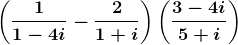(xii) (5 +√2i) / (1-√2i)

Solution:

(i) (1 + i) (1 + 2i)

Let us simplify and express in the standard form of (a + ib),

(1 + i) (1 + 2i) = (1+i)(1+2i)

= 1(1+2i)+i(1+2i)

= 1+2i+i+2i2

= 1+3i+2(-1) [since, i2 = -1]

= 1+3i-2

= -1+3i

∴ The values of a, b are -1, 3.

(ii) (3 + 2i) / (-2 + i)

Let us simplify and express in the standard form of (a + ib),

(3 + 2i) / (-2 + i) = [(3 + 2i) / (-2 + i)] × (-2-i) / (-2-i) [multiply and divide with (-2-i)]

= [3(-2-i) + 2i (-2-i)] / [(-2)2 – (i)2]

= [-6 -3i – 4i -2i2] / (4-i2)

= [-6 -7i -2(-1)] / (4 – (-1)) [since, i2 = -1]

= [-4 -7i] / 5

∴ The values of a, b are -4/5, -7/5

(iii) 1/(2 + i)2

Let us simplify and express in the standard form of (a + ib),

1/(2 + i)2 = 1/(22 + i2 + 2(2) (i))

= 1/ (4 – 1 + 4i) [since, i2 = -1]

= 1/(3 + 4i) [multiply and divide with (3 – 4i)]

= 1/(3 + 4i) × (3 – 4i)/ (3 – 4i)]

= (3-4i)/ (32 – (4i)2)

= (3-4i)/ (9 – 16i2)

= (3-4i)/ (9 – 16(-1)) [since, i2 = -1]

= (3-4i)/25

∴ The values of a, b are 3/25, -4/25

(iv) (1 – i) / (1 + i)

Let us simplify and express in the standard form of (a + ib),

(1 – i) / (1 + i) = (1 – i) / (1 + i) × (1-i)/(1-i) [multiply and divide with (1-i)]

= (12 + i2 – 2(1)(i)) / (12 – i2)

= (1 + (-1) -2i) / (1 – (-1))

= -2i/2

= -i

∴ The values of a, b are 0, -1

(v) (2 + i)3 / (2 + 3i)

Let us simplify and express in the standard form of (a + ib),

(2 + i)3 / (2 + 3i) = (23 + i3 + 3(2)2(i) + 3(i)2(2)) / (2 + 3i)

= (8 + (i2.i) + 3(4)(i) + 6i2) / (2 + 3i)

= (8 + (-1)i + 12i + 6(-1)) / (2 + 3i)

= (2 + 11i) / (2 + 3i)

[multiply and divide with (2-3i)]

= (2 + 11i)/(2 + 3i) × (2-3i)/(2-3i)

= [2(2-3i) + 11i(2-3i)] / (22 – (3i)2)

= (4 – 6i + 22i – 33i2) / (4 – 9i2)

= (4 + 16i – 33(-1)) / (4 – 9(-1)) [since, i2 = -1]

= (37 + 16i) / 13

∴ The values of a, b are 37/13, 16/13

(vi) [(1 + i) (1 +√3i)] / (1 – i)

Let us simplify and express in the standard form of (a + ib),

[(1 + i) (1 +√3i)] / (1 – i) = [1(1+√3i) + i(1+√3i)] / (1-i)

= (1 + √3i + i + √3i2) / (1 – i)

= (1 + (√3+1)i + √3(-1)) / (1-i) [since, i2 = -1]

= [(1-√3) + (1+√3)i] / (1-i)

[multiply and divide with (1+i)]

= [(1-√3) + (1+√3)i] / (1-i) × (1+i)/(1+i)

= [(1-√3) (1+i) + (1+√3)i(1+i)] / (12 – i2)

= [1-√3+ (1-√3)i + (1+√3)i + (1+√3)i2] / (1-(-1)) [since, i2 = -1]

= [(1-√3)+(1-√3+1+√3)i+(1+√3)(-1)] / 2

= (-2√3 + 2i) / 2

= -√3 + i

∴ The values of a, b are -√3, 1

(vii) (2 + 3i) / (4 + 5i)

Let us simplify and express in the standard form of (a + ib),

(2 + 3i) / (4 + 5i) = [multiply and divide with (4-5i)]

= (2 + 3i) / (4 + 5i) × (4-5i)/(4-5i)

= [2(4-5i) + 3i(4-5i)] / (42 – (5i)2)

= [8 – 10i + 12i – 15i2] / (16 – 25i2)

= [8+2i-15(-1)] / (16 – 25(-1)) [since, i2 = -1]

= (23 + 2i) / 41

∴ The values of a, b are 23/41, 2/41

(viii) (1 – i)3 / (1 – i3)

Let us simplify and express in the standard form of (a + ib),

(1 – i)3 / (1 – i3) = [13 – 3(1)2i + 3(1)(i)2 – i3] / (1-i2.i)

= [1 – 3i + 3(-1)-i2.i] / (1 – (-1)i) [since, i2 = -1]

= [-2 – 3i – (-1)i] / (1+i)

= [-2-4i] / (1+i)

[Multiply and divide with (1-i)]

= [-2-4i] / (1+i) × (1-i)/(1-i)

= [-2(1-i)-4i(1-i)] / (12 – i2)

= [-2+2i-4i+4i2] / (1 – (-1))

= [-2-2i+4(-1)] /2

= (-6-2i)/2

= -3 – i

∴ The values of a, b are -3, -1

(ix) (1 + 2i)-3

Let us simplify and express in the standard form of (a + ib),

(1 + 2i)-3 = 1/(1 + 2i)3

= 1/(13+3(1)2 (2i)+2(1)(2i)2 + (2i)3)

= 1/(1+6i+4i2+8i3)

= 1/(1+6i+4(-1)+8i2.i) [since, i2 = -1]

= 1/(-3+6i+8(-1)i) [since, i2 = -1]

= 1/(-3-2i)

= -1/(3+2i)

[Multiply and divide with (3-2i)]

= -1/(3+2i) × (3-2i)/(3-2i)

= (-3+2i)/(32 – (2i)2)

= (-3+2i) / (9-4i2)

= (-3+2i) / (9-4(-1))

= (-3+2i) /13

∴ The values of a, b are -3/13, 2/13

(x) (3 – 4i) / [(4 – 2i) (1 + i)]

Let us simplify and express in the standard form of (a + ib),

(3 – 4i) / [(4 – 2i) (1 + i)] = (3-4i)/ [4(1+i)-2i(1+i)]

= (3-4i)/ [4+4i-2i-2i2]

= (3-4i)/ [4+2i-2(-1)] [since, i2 = -1]

= (3-4i)/ (6+2i)

[Multiply and divide with (6-2i)]

= (3-4i)/ (6+2i) × (6-2i)/(6-2i)

= [3(6-2i)-4i(6-2i)] / (62 – (2i)2)

= [18 – 6i – 24i + 8i2] / (36 – 4i2)

= [18 – 30i + 8 (-1)] / (36 – 4 (-1)) [since, i2 = -1]

= [10-30i] / 40

= (1 – 3i) / 4

∴ The values of a, b are 1/4, -3/4

(xi)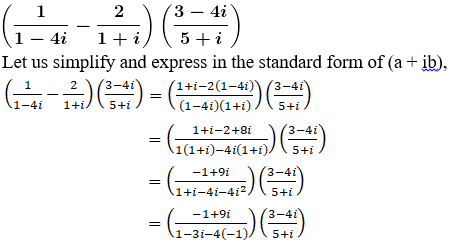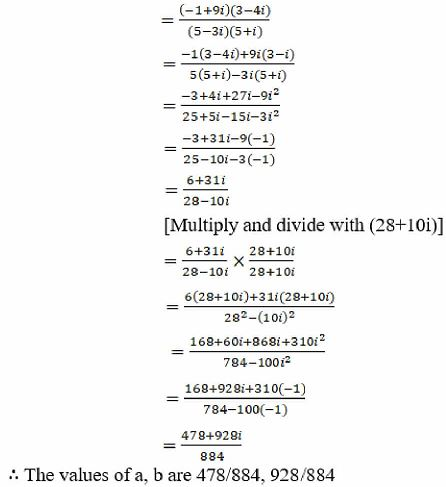(xii) (5 +√2i) / (1-√2i)

Let us simplify and express in the standard form of (a + ib),

(5 +√2i) / (1-√2i) = [Multiply and divide with (1+2i)]

= (5 +√2i) / (1-√2i) × (1+2i)/(1+2i)

= [5(1+2i) + 2i(1+2i)] / (12 – (2)2)

= [5+52i + 2i + 2i2] / (1 – 2i2)

= [5 + 62i + 2(-1)] / (1-2(-1)) [since, i2 = -1]

= [3+62i]/3

= 1+ 22i

∴ The values of a, b are 1, 22

2. Find the real values of x and y, if

(i) (x + iy) (2 – 3i) = 4 + i

(ii) (3x – 2i y) (2 + i)2 = 10(1 + i)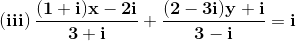(iv) (1 + i) (x + iy) = 2 – 5i

Solution:

(i) (x + iy) (2 – 3i) = 4 + i

Given:

(x + iy) (2 – 3i) = 4 + i

Let us simplify the expression we get,

x(2 – 3i) + iy(2 – 3i) = 4 + i

2x – 3xi + 2yi – 3yi2 = 4 + i

2x + (-3x+2y)i – 3y (-1) = 4 + i [since, i2 = -1]

2x + (-3x+2y)i + 3y = 4 + i [since, i2 = -1]

(2x+3y) + i(-3x+2y) = 4 + i

Equating Real and Imaginary parts on both sides, we get

2x+3y = 4… (i)

And -3x+2y = 1… (ii)

Multiply (i) by 3 and (ii) by 2 and add

On solving we get,

6x – 6x – 9y + 4y = 12 + 2

13y = 14

y = 14/13

Substitute the value of y in (i) we get,

2x+3y = 4

2x + 3(14/13) = 4

2x = 4 – (42/13)

= (52-42)/13

2x = 10/13

x = 5/13

x = 5/13, y = 14/13

∴ The real values of x and y are 5/13, 14/13

(ii) (3x – 2i y) (2 + i)2 = 10(1 + i)

Given:

(3x – 2iy) (2+i)2 = 10(1+i)

(3x – 2yi) (22+i2+2(2)(i)) = 10+10i

(3x – 2yi) (4 + (-1)+4i) = 10+10i [since, i2 = -1]

(3x – 2yi) (3+4i) = 10+10i

Let us divide with 3+4i on both sides we get,

(3x – 2yi) = (10+10i)/(3+4i)

= Now multiply and divide with (3-4i)

= [10(3-4i) + 10i(3-4i)] / (32 – (4i)2)

= [30-40i+30i-40i2] / (9 – 16i2)

= [30-10i-40(-1)] / (9-16(-1))

= [70-10i]/25

Now, equating Real and Imaginary parts on both sides we get

3x = 70/25 and -2y = -10/25

x = 70/75 and y = 1/5

x = 14/15 and y = 1/5

∴ The real values of x and y are 14/15, 1/5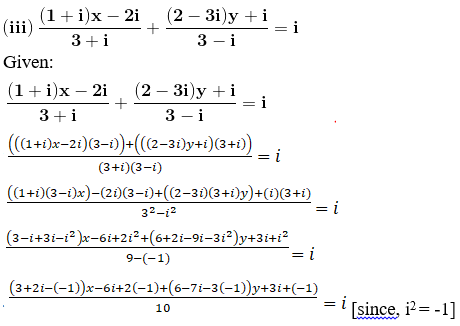(4+2i) x-3i-3 + (9-7i)y = 10i

(4x+9y-3) + i(2x-7y-3) = 10i

Now, equating Real and Imaginary parts on both sides we get,

4x+9y-3 = 0 … (i)

And 2x-7y-3 = 10

2x-7y = 13 … (ii)

Multiply (i) by 7 and (ii) by 9 and add

On solving these equations we get

28x + 18x + 63y – 63y = 117 + 21

46x = 117 + 21

46x = 138

x = 138/46

= 3

Substitute the value of x in (i) we get,

4x+9y-3 = 0

9y = -9

y = -9/9

= -1

x = 3 and y = -1

∴ The real values of x and y are 3 and -1

(iv) (1 + i) (x + iy) = 2 – 5i

Given:

(1 + i) (x + iy) = 2 – 5i

Divide with (1+i) on both the sides we get,

(x + iy) = (2 – 5i)/(1+i)

Multiply and divide by (1-i)

= (2 – 5i)/(1+i) × (1-i)/(1-i)

= [2(1-i) – 5i (1-i)] / (12 – i2)

= [2 – 7i + 5(-1)] / 2 [since, i2 = -1]

= (-3-7i)/2

Now, equating Real and Imaginary parts on both sides we get

x = -3/2 and y = -7/2

∴ Thee real values of x and y are -3/2, -7/2

3. Find the conjugates of the following complex numbers:

(i) 4 – 5i

(ii) 1 / (3 + 5i)

(iii) 1 / (1 + i)

(iv) (3 – i)2 / (2 + i)

(v) [(1 + i) (2 + i)] / (3 + i)

(vi) [(3 – 2i) (2 + 3i)] / [(1 + 2i) (2 – i)]

Solution:

(i) 4 – 5i

Given:

4 – 5i

We know the conjugate of a complex number (a + ib) is (a – ib)

So,

∴ The conjugate of (4 – 5i) is (4 + 5i)

(ii) 1 / (3 + 5i)

Given:

1 / (3 + 5i)

Since the given complex number is not in the standard form of (a + ib)

Let us convert to standard form by multiplying and dividing with (3 – 5i)

We get,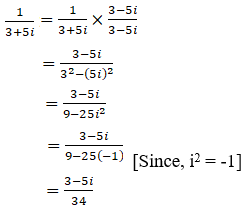We know the conjugate of a complex number (a + ib) is (a – ib)

So,

∴ The conjugate of (3 – 5i)/34 is (3 + 5i)/34

(iii) 1 / (1 + i)

Given:

1 / (1 + i)

Since the given complex number is not in the standard form of (a + ib)

Let us convert to standard form by multiplying and dividing with (1 – i)

We get,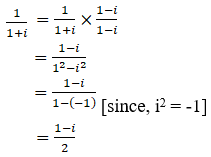We know the conjugate of a complex number (a + ib) is (a – ib)

So,

∴ The conjugate of (1-i)/2 is (1+i)/2

(iv) (3 – i)2 / (2 + i)

Given:

(3 – i)2 / (2 + i)

Since the given complex number is not in the standard form of (a + ib)

Let us convert to standard form,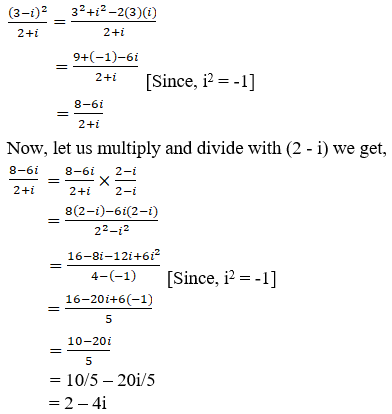We know the conjugate of a complex number (a + ib) is (a – ib)

So,

∴ The conjugate of (2 – 4i) is (2 + 4i)

(v) [(1 + i) (2 + i)] / (3 + i)

Given:

[(1 + i) (2 + i)] / (3 + i)

Since the given complex number is not in the standard form of (a + ib)

Let us convert to standard form,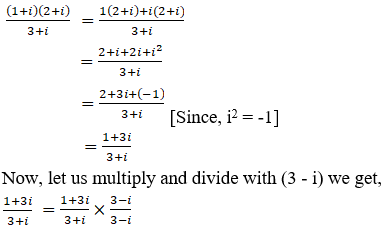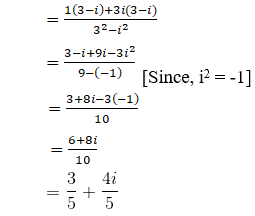We know the conjugate of a complex number (a + ib) is (a – ib)

So,

∴ The conjugate of (3 + 4i)/5 is (3 – 4i)/5

(vi) [(3 – 2i) (2 + 3i)] / [(1 + 2i) (2 – i)]

Given:

[(3 – 2i) (2 + 3i)] / [(1 + 2i) (2 – i)]

Since the given complex number is not in the standard form of (a + ib)

Let us convert to standard form,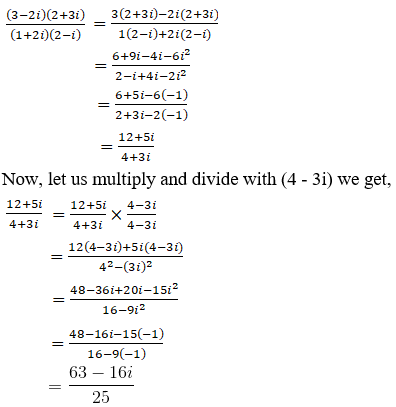We know the conjugate of a complex number (a + ib) is (a – ib)

So,

∴ The conjugate of (63 – 16i)/25 is (63 + 16i)/25

4. Find the multiplicative inverse of the following complex numbers:

(i) 1 – i

(ii) (1 + i √3)2

(iii) 4 – 3i

(iv) √5 + 3i

Solution:

(i) 1 – i

Given:

1 – i

We know the multiplicative inverse of a complex number (Z) is Z-1 or 1/Z

So,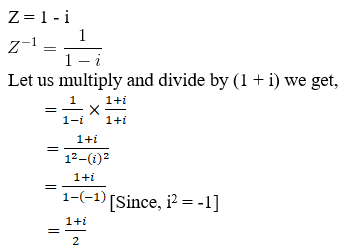∴ The multiplicative inverse of (1 – i) is (1 + i)/2

(ii) (1 + i √3)2

Given:

(1 + i √3)2

Z = (1 + i √3)2

= 12 + (i √3)2 + 2 (1) (i√3)

= 1 + 3i2 + 2 i√3

= 1 + 3(-1) + 2 i√3 [since, i2 = -1]

= 1 – 3 + 2 i√3

= -2 + 2 i√3

We know the multiplicative inverse of a complex number (Z) is Z-1 or 1/Z

So,

Z = -2 + 2 i√3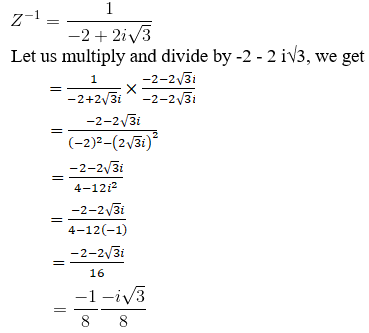∴ The multiplicative inverse of (1 + i√3)2 is (-1-i√3)/8

(iii) 4 – 3i

Given:

4 – 3i

We know the multiplicative inverse of a complex number (Z) is Z-1 or 1/Z

So,

Z = 4 – 3i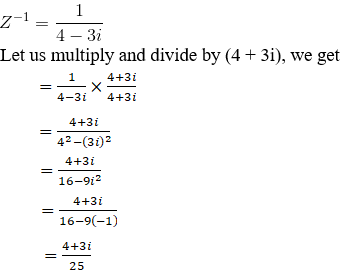∴ The multiplicative inverse of (4 – 3i) is (4 + 3i)/25

(iv) √5 + 3i

Given:

√5 + 3i

We know the multiplicative inverse of a complex number (Z) is Z-1 or 1/Z

So,

Z = √5 + 3i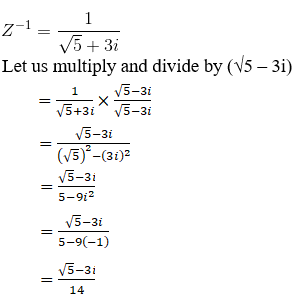∴ The multiplicative inverse of (√5 + 3i) is (√5 – 3i)/14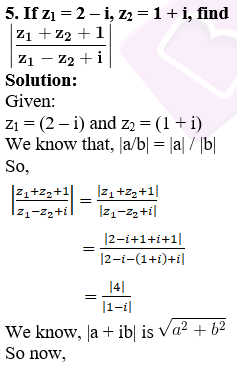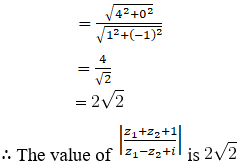6. If z1 = (2 – i), z2 = (-2 + i), find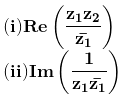Solution:

Given:

z1 = (2 – i) and z2 = (-2 + i)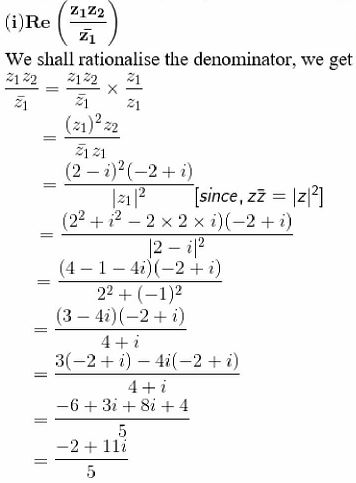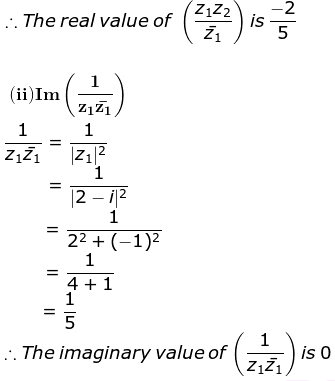7. Find the modulus of [(1 + i)/(1 – i)] – [(1 – i)/(1 + i)]

Solution:

Given:

[(1 + i)/(1 – i)] – [(1 – i)/(1 + i)]

So,

Z = [(1 + i)/(1 – i)] – [(1 – i)/(1 + i)]

Let us simplify, we get

= [(1+i) (1+i) – (1-i) (1-i)] / (12 – i2)

= [12 + i2 + 2(1)(i) – (12 + i2 – 2(1)(i))] / (1 – (-1)) [Since, i2 = -1]

= 4i/2

= 2i

We know that for a complex number Z = (a+ib) it’s magnitude is given by |z| = (a2 + b2)

So,

|Z| = (02 + 22)

= 2

∴ The modulus of [(1 + i)/(1 – i)] – [(1 – i)/(1 + i)] is 2.

8. If x + iy = (a+ib)/(a-ib), prove that x2 + y2 = 1

Solution:

Given:

x + iy = (a+ib)/(a-ib)

We know that for a complex number Z = (a+ib) it’s magnitude is given by |z| = (a2 + b2)

So,

|a/b| is |a| / |b|

Applying Modulus on both sides we get,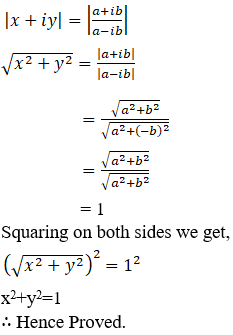9. Find the least positive integral value of n for which [(1+i)/(1-i)]n is real.

Solution:

Given:

[(1+i)/(1-i)]n

Z = [(1+i)/(1-i)]n

Now let us multiply and divide by (1+i), we get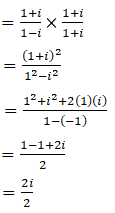= i [which is not real]

For n = 2, we have

[(1+i)/(1-i)]2 = i2

= -1 [which is real]

So, the smallest positive integral ‘n’ that can make [(1+i)/(1-i)]n real is 2.

∴ The smallest positive integral value of ‘n’ is 2.

10. Find the real values of θ for which the complex number (1 + i cos θ) / (1 – 2i cos θ) is purely real.

Solution:

Given:

(1 + i cos θ) / (1 – 2i cos θ)

Z = (1 + i cos θ) / (1 – 2i cos θ)

Let us multiply and divide by (1 + 2i cos θ)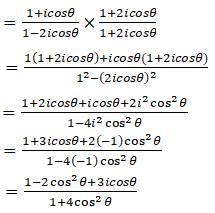For a complex number to be purely real, the imaginary part should be equal to zero.

So,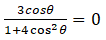3cos θ = 0 (since, 1 + 4cos2θ ≥ 1)

cos θ = 0

cos θ = cos π/2

θ = [(2n+1)π] / 2, for n ∈ Z

= 2nπ ± π/2, for n ∈ Z

∴ The values of θ to get the complex number to be purely real is 2nπ ± π/2, for n ∈ Z

11. Find the smallest positive integer value of n for which (1+i) n / (1-i) n-2 is a real number.

Solution:

Given:

(1+i) n / (1-i) n-2

Z = (1+i) n / (1-i) n-2

Let us multiply and divide by (1 – i)2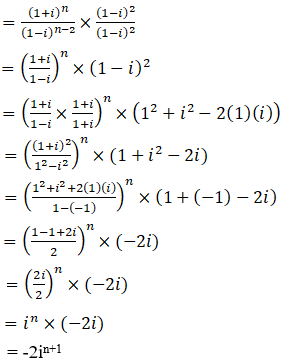For n = 1,

Z = -2i1+1

= -2i2

= 2, which is a real number.

∴ The smallest positive integer value of n is 1.

12. If [(1+i)/(1-i)]3 – [(1-i)/(1+i)]3 = x + iy, find (x, y)

Solution:

Given:

[(1+i)/(1-i)]3 – [(1-i)/(1+i)]3 = x + iy

Let us rationalize the denominator, we get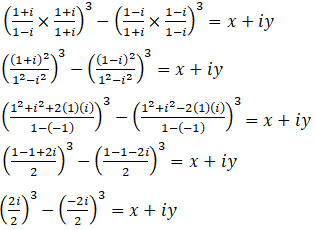i3–(-i)3 = x + iy

2i3 = x + iy

2i2.i = x + iy

2(-1)I = x + iy

-2i = x + iy

Equating Real and Imaginary parts on both sides we get

x = 0 and y = -2

∴ The values of x and y are 0 and -2.

13. If (1+i)2 / (2-i) = x + iy, find x + y

Solution:

Given:

(1+i)2 / (2-i) = x + iy

Upon expansion we get,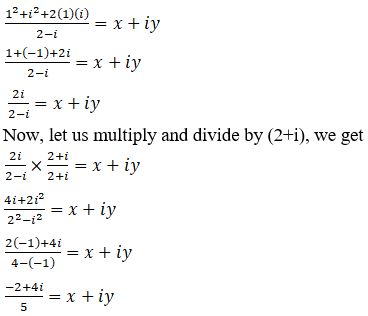Let us equate real and imaginary parts on both sides we get,

x = -2/5 and y = 4/5

so,

x + y = -2/5 + 4/5

= (-2+4)/5

= 2/5

∴ The value of (x + y) is 2/5